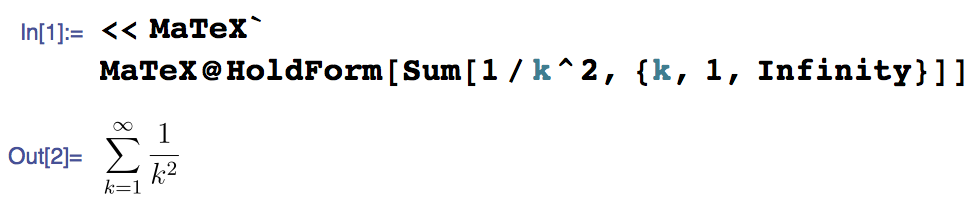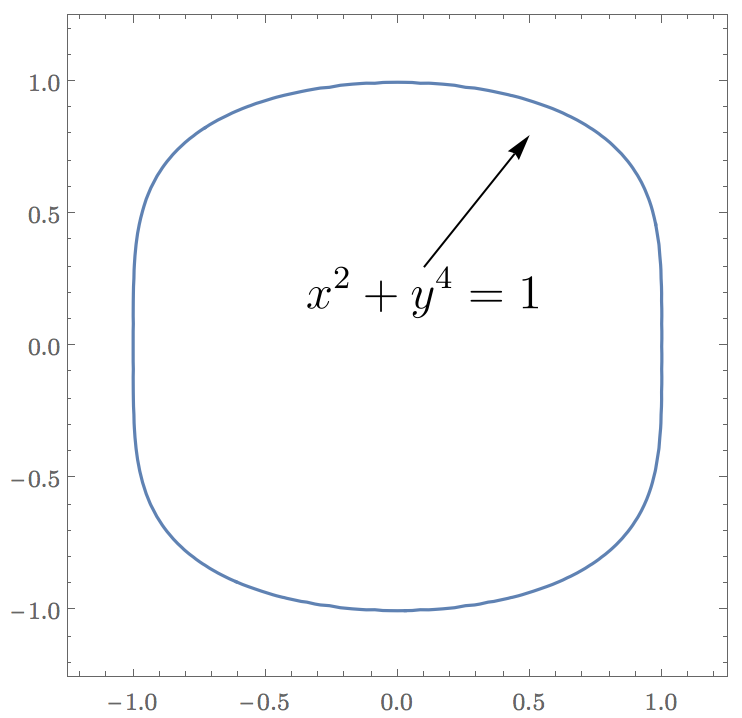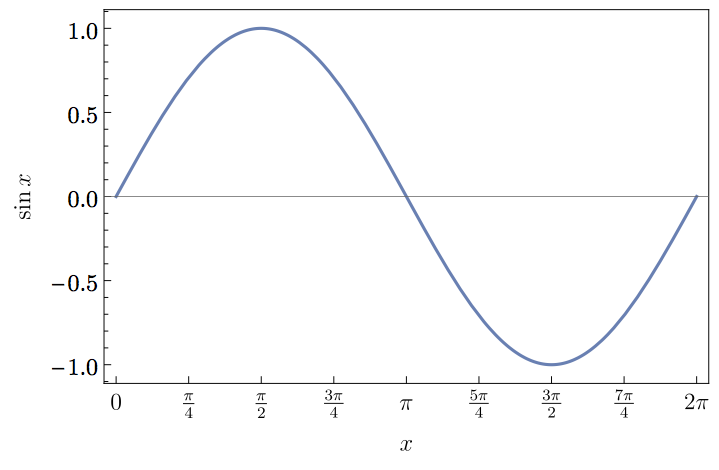# LaTeX typesetting in Mathematica

If you are looking for documentation on MaTeX, simply search for “matex” in Mathematica’s documentation center!

Mathematica is an excellent and flexible visualization tool, and even supports displaying complex mathematical formulae. However, its typesetting quality is not on par with $$\mathsf{\LaTeX}$$. The visual style is not a good match for inclusion in LaTeX documents either. To improve the quality of my figures, I wrote a small Mathematica package that makes it easy to use LaTeX-generated labels: MaTeX.

Update: The post below has been updated for MaTeX version 1.7.9. The changelog is available on GitHub.

There are already several solutions for this problem, such as PSTricks or drawing axes and labels with PGFPlots. None of these solutions make it easy to use a Mathematica-centric workflow though. MaTeX makes generating LaTeX-typeset expressions as simple asI originally wrote these functions for my own needs, but seeing that others may be interested in them too, I wrapped them up into a package. Feel free to leave comments or suggestion below!

## Installation

In Mathematica 11.3 or later, simply evaluate the following:

ResourceFunction["MaTeXInstall"][]


In earlier Mathematica versions that do not support resource functions, download the latest release, distributed as a .paclet file, and install it using the PacletInstall Mathematica function:

Needs["PacletManager"]
PacletInstall["/full/path/to/MaTeX-1.7.9.paclet"]


See the instructions on the GitHub page for more details, and for an automatic installation and upgrade script.

You will also need:

• A TeX system that includes pdflatex with the standalone, exscale and lmodern packages. Both TeX Live and MiKTeX should work.
• Ghostscript 9.15 or later. On OS X, MacTeX 2015 includes a compatible version of Ghostscript. If you use an older TeX distribution on OS X, get a recent Ghostscript from Richard Koch’s page.

MaTeX can be loaded as usual,

<<MaTeX


When loading MaTeX for the first time, it will try to automatically detect TeX and Ghostscript. If this fails, it will display instructions on configuring the location of the pdflatex and gs executables using the ConfigureMaTeX command. As an example, on my OS X system I needed to use the following configuration:

ConfigureMaTeX["pdfLaTeX" -> "/Library/TeX/texbin/pdflatex", "Ghostscript" -> "/usr/local/bin/gs"]


The existing configuration can always be queried using the command ConfigureMaTeX[].

Notes:

• On Windows use the c-suffixed command line Ghostscript executable, i.e. gswin64c.exe instead of gswin64.exe.

• If you prefer, you can use xelatex instead of pdflatex. Unlike plain pdfLaTeX, XeLaTeX can load any installed system font.

• The auto-detection can be re-run any time using MaTeXDeveloperResestConfiguration[]. Warning: This will discard the existing configuration.

• Some users report that the documentation becomes available only after restarting the Mathematica front end. The documentation can be accessed by selecting Wolfram Documentation from the Help menu, then searching for “matex” or “MaTeX”.

MaTeX should now be ready to use. Test it using MaTeX["x^2"].

## Usage examples

Note: Many more usage examples are available in the integrated documentation. Search for “MaTeX” in Mathematica’s Documentation Center to access them.

First we need to load MaTeX:

<<MaTeX


My primary use for it is creating figure annotations, as below:

texStyle = {FontFamily -> "Latin Modern Roman", FontSize -> 12};

ContourPlot[x^2 + y^4 == 1, {x, -1.2, 1.2}, {y, -1.2, 1.2},
BaseStyle -> texStyle,
Epilog -> {
Arrow[{{0.1, 0.3}, {0.5, 0.80}}],
Inset[MaTeX["x^2+y^4=1", Magnification -> 2], {0.1, 0.3}, Scaled[{0.5, 1}]]
}]Here I used the Latin Modern font for tick labels for visual consistency with the MaTeX/LaTeX output. Note that on different operating systems it may be necessary to refer to the same font with different names.

We can also use MaTeX to generate beautifully typeset frame labels and frame ticks. Mathematica’s default frame and axes style is dark grey, while MateX outputs black. The BlackFrame style below makes the frames black too for consistency.

Plot[Sin[x], {x, 0, 2 Pi},
Frame -> True, FrameStyle -> BlackFrame,
FrameTicks -> {{Automatic, None},
{Table[{x, MaTeX[x, "DisplayStyle" -> False]}, {x, Pi/4 Range[0, 8]}], None}},
FrameLabel -> MaTeX /@ {"x", "\\sin x"},
BaseStyle -> texStyle]The code above will run slowly because processing each tick label involves a separate and costly call to LaTeX. Since MaTeX 1.6, we can use much faster batch processing by applying MaTeX to a list of expressions:

Plot[Sin[x], {x, 0, 2 Pi},
Frame -> True, FrameStyle -> BlackFrame,
FrameTicks -> {{Automatic, None},
{With[{ticks = Pi/4 Range[0, 8]}, Thread[{ticks, MaTeX[ticks, "DisplayStyle" -> False]}]], None}},
FrameLabel -> MaTeX @ {"x", "\\sin x"},
BaseStyle -> texStyle]


The MaTeX function can be used either with a string containing math-mode LaTeX code, or with an arbitrary Mathematica expression. It will automatically apply TeXForm to non-string expressions.

When writing TeX code in Mathematica strings, remember to always escape backslashes. Thus \sum must be written as "\\sum". (A trick to dodge the need to escape backslashes with MaTeX 1.7.3 or later is shown in the Neat Examples section of the MaTeX documentation page.)

### Options

• "DisplayStyle".  By default display style is used. Use "DisplayStyle" -> False for inline style.

Inline formatting looks like $$\sum_{k=1}^\infty \frac{1}{k^2} = \frac{\pi^2}{6}$$. Display style looks like $$\displaystyle\sum_{k=1}^\infty \frac{1}{k^2} = \frac{\pi^2}{6}$$.

• FontSize.  Use it to set the font size. Note that LaTeX uses different glyph shapes for different font sizes to improve readability. Depending on the font used, only standard sizes may be available with this option. Use Magnification instead for proportional scaling.

• "Preamble".  This is a list of lines to be included in the LaTeX preamble. The default is {}, i.e. empty.

This option is most convenient to set permanently for the session, for example:

SetOptions[MaTeX, "Preamble" -> {"\\usepackage{color,txfonts}"}]
MaTeX["\\color{red}\\sqrt{x}"]

• "BasePreamble".  Will be included just before "Preamble" in the LaTeX preamble. The default value is {"\\usepackage{lmodern,exscale}", "\\usepackage{amsmath,amssymb}"}. The AMS packages are included by default because they may be required for compiling the output of Mathematica’s TeXForm, while lmodern provides vector fonts and flexible font sizing. On most systems "\\usepackage{lmodern,exscale}" can be omitted.

The reasoning for having a second preamble option is that most for applications it is desirable to keep the LaTeX packages that are in "BasePreamble" by default. "Preamble" can be set without having to pay special attention to keeping these, as they are already in "BasePreamble".

• Magnification.  Set a scaling factor for MaTeX’s output. Magnification scales the result proportionally, unlike FontSize, which will use different glyph shapes for small text.

• ContentPadding.  With ContentPadding -> True (the default) the height of the output will be at least one line height. Setting it to False will crop the output as tight around the content as possible (but note that MaTeX always leaves a margin of 1 pt).

• LineSpacingLineSpacing -> {c, n} sets the line height to c times the FontSize plus n points. The default is {1.2, 0}, i.e. 1.2 times the font size. The method for computing the line height has changed in MaTeX 1.2.0. To restore the old behaviour, use LineSpacing -> {0, 14.4}.

### Performance

MaTeX needs to call pdflatex for every LaTeX expression it generates. Each call might take as long as a second, which can be annoyingly slow when using MaTeX on many small expression, e.g. creating tick labels. As a partial remedy for this, MaTeX caches each result, so a second call with the same TeX code should be instantaneous.

The maximum number of expressions to be cached can be controlled using ConfigureMaTeX["CacheSize" -> ...]. Set "CacheSize" to 0 to disable caching or Infinity to prevent limiting the number of cached results. ClearMaTeXCache[] will clear the cache.

Since version 1.6, MaTeX can process a list of expressions with a single run of LaTeX. Structuring your code in a way to allow batch-processing of lists can improve performance significantly. This can be useful e.g. when generating tick labels.

Note: pdflatex performs slightly better than xelatex. On my system, one call to MaTeX takes ~0.33 s with pdflatex and ~0.55 s with xelatex.

## How does it work?

MaTeX uses pdflatex and the standalone document class to create PDF files from TeX code. The height of the PDF files is ensured to be at least the line height by including a \strut (unless ContentPadding -> False is used). Mathematica can import PDF files, but it can only interpret simple ones correctly. To avoid problems, all font glyphs are converted to outline curves using Ghostscript before import. This capability is new in Ghostscript 9.15, hence the version requirement.

## Support and Troubleshooting

If MaTeX does not produce the output you expect, be sure to check the Possible Issues section in the MaTeX documentation page in Mathematica’s Documentation Center. Simply open the Documentation Center form the Help menu, and search for “MaTeX”.

##### Get::noopen: Cannot open MaTeX. message when loading MaTeX

MaTeX requires Mathematica 10.0 or later. This message may be shown in earlier versions when trying to load the package.

If you see this message with a recent Mathematica version, it means that MaTeX is not installed.

##### Cannot typeset systems of equations

MaTeX only supports LaTeX that is valid in inline math mode (i.e. $...$). This means that certain environments, such as eqnarray or split will not work. aligned does work however.

MaTeX["
\\begin{aligned}
x+y&=z \\\\
u+v&=w
\\end{aligned}
"]

##### Cannot typeset multiline equations with split environment

MaTeX always uses the inline math environment, even for producing display style output. This means that certain environments, such as the split environment from the amsmath do not work. You may see an error such as

! Package amsmath Error: \begin{split} won't work here.

A practical workaround is to use the aligned environment, which does work in inline math. Example:

MaTeX["
\\begin{aligned}
(1+x)^{10} = {} & x^{10} + 10 x^9 + 45 x^8 + 120 x^7 + 210 x^6 + {}\\\\
& + 252 x^5 + 210 x^4 + 120 x^3 + 45 x^2 + 10 x + 1
\\end{aligned}
"]


Another option is to use multlined from the mathtools package.

##### New versions of MaTeX use different margins and now my old figures don’t look good anymore!

The method for computing the line height has changed in MaTeX 1.2.0. To restore the old behaviour (i.e. to re-run code written with older versions of MaTeX), use LineSpacing -> {0, 14.4}.

##### New versions of MaTeX do not treat lists as a single unit anymore

MaTeX 1.6 and later automatically thread over lists. To force treating the list as a single unit, use

MaTeX[TeXForm[list]]

##### MaTeX no longer works after upgrading to OS X 10.11 El Capitan

If you use MacTeX on OS X El Capitan or later, the path to pdflatex or xelatex must be set as

ConfigureMaTeX["pdfLaTeX" -> "/Library/TeX/texbin/pdflatex"]


If you used the path /usr/texbin/pdflatex on OS X 10.10 or earlier, it will no longer work on 10.11. You must reconfigure MaTeX with the new path. Please see the explanation here.

The path to Ghostscript is unchanged, /usr/local/bin/gs.

##### “MaTeX::texerr : Error while running LaTeX” message

There may be several causes for this message.

If it only happens with certain bits of TeX code, but not others, then it indicates that there is an error in your LaTeX code. MaTeX attempts to extract the relevant error message from LaTeX’s console output and report it, but it does not always succeed in this. To make debugging such situations easier, MaTeX offers the "LogFileFunction" and "TeXFileFunction" options (since version 1.3.0). These are applied to the contents of the LaTeX log file and to the generated LaTeX code, respectively.

Use them like this:

MaTeX["x^", "LogFileFunction" -> Print, "TeXFileFunction" -> Print]


The provided functions are called only if MaTeX is not using a previously cached result. To force calling them, clear the cache using ClearMaTeXCache[].

If this error appears with any input to MaTeX, even with a simple example such as MaTeX["x^2"], then there may be a problem with your setup or MaTeX may be incompatible with your system. Check for the following:

• Do you have all required LaTeX packages installed? MaTeX requires standalone, lmodern, exscale, amsmath and amssymb. All these are part of most TeX distributions, but may not get installed if choosing the smallest installation option. The "LogFileFunction" option (described above) can help in diagnosing missing packages.

• If you use OS X or Windows (with certain TeX distributions only), make sure that the name of the pdflatex (or xelatex) executable is spelt in the correct case in ConfigureMaTeX. It must be lowercase.

Examples for OS X:

ConfigureMaTeX["pdfLaTeX" -> "/Library/TeX/texbin/pdflatex"] is correct and will work provided that this is indeed the location of pdflatex on your system.

ConfigureMaTeX["pdfLaTeX" -> "/Library/TeX/texbin/pdfLaTeX"] is wrong and will trigger the error.

OS X uses a case-preserving but case-insensitive file system by default. However, some programs with a UNIX heritage may not work correctly unless they are invoked with the case-correct spelling. pdflatex is a notable example because it determines the format file to use based on the name it is called with. The problem may also affect some TeX distributions on Windows, such as TeX Live in Cygwin.

• If you use a 32-bit version of Mathematica 10.0 for Windows, MaTeX will not work. This is due to a bug in this version of Mathematica. As a workaround, use a 64-bit version of Mathematica or upgrade to a later 10.x version. Version 10.3.1 reportedly works correctly. I have no information about 10.1 and 10.2.

If the error shows Undefined control sequence: \documentclass, then MaTeX was probably configured to use pdftex instead of pdflatex. Configure it to use pdflatex (or another compatible flavour of LaTeX, such as xelatex).

##### Loading MaTeX or using the MaTeX function triggers a RunProcess::pnfd error on Windows

This may happen for two reasons:

1. If MaTeX’s working directory contains non-ASCII characters in its name (such as é, ő, α, 中, etc.), RunProcess will fail in some versions of Mathematica for Windows. This is a bug in Mathematica. Earlier versions of MaTeX contained a partial workaround for this issue, however, that workaround had the potential to cause problems, therefore it was removed. Since MaTeX 1.7.3, it is possible to set MaTeX’s working directory manually. Create a directory which only has ASCII characters in its path, e.g. C:\temp\MateX, and use ConfigureMaTeX["WorkingDirectory"-> "C:\\temp\\MaTeX"] to instruct MaTeX to use it. The setting will be saved for future sessions. To revert to the default behaviour, set ConfigureMaTeX["WorkingDirectory"-> Automatic]. See the documentation of ConfigureMaTeX for more information.

2. The paths to pdfLaTeX and Ghostscript use / as the path separator. Please use ConfigureMaTeX and change the paths to use backslashes (\) as the separator. Remember that backslashes must be escaped within Mathematica strings, so a path string will look something like "C:\\texlive\\2014\\bin\\win64\\pdflatex.exe".

##### MaTeX doesn’t work when configured to use lualatex

When using LuaTeX 0.85 or later, it may be required to use the luatex85 package. The package documentation explains why this is needed.

Add "\\usepackage{luatex85}" to the "Preamble" or "BasePreamble" options.

#### MaTeX still doesn’t work!

Download this notebook, and evaluate it by following the instructions at the top precisely. This will record some information that I can use to debug the problem. Save the notebook and send it to me along with a complete description of the problems you are seeing.